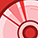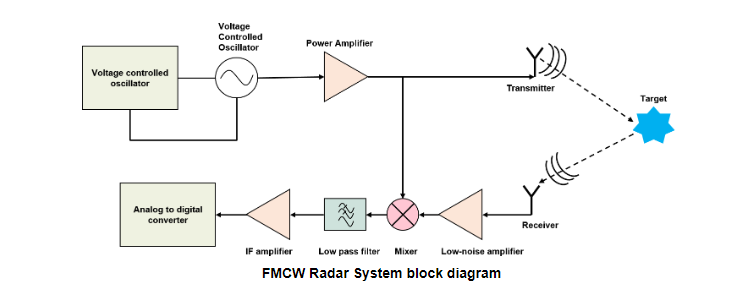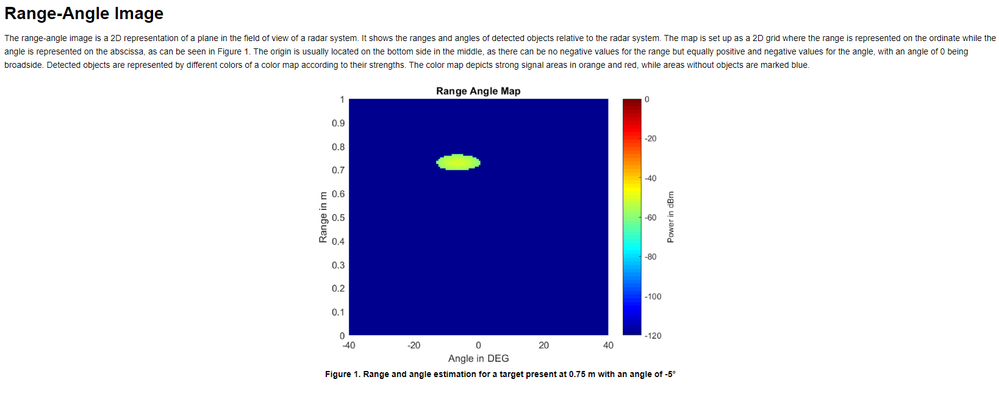Level 1Level 1

# raw data question

Hello i use Radar fusion GUI do recording and get the xx.raw.txt data.

# Are_Rx_Antennas_Interleaved = 1
# Modulation_Type_Enum = 1
# Chirp_Shape_Enum = 0
# Lower_RF_Frequency_kHz = 6.0238e+07
# Upper_RF_Frequency_kHz = 6.0762e+07
# Sampling_Frequency_kHz = 1000
# Data_Format_Enum = 0
# Chirps_per_Frame = 64
# Samples_per_Chirp = 64
# Samples_per_Frame = 12288
# Chirp_Time_sec = 6.89875e-05
# Pulse_Repetition_Time_sec = 0.000505637
# Frame_Period_sec = 0.1

# Frame_Number = 19491
0.4884
0.484005
0.477167
0.47326
0.477656

i know the data (0.484005) from analog signal do ADC convet it to value in [0, 1] in default setting.

while i have some question about the raw data.

=> first question about the data, what does the value 0.484005 or 0.477167 means?

does it means the  amplitude of the size in analog signal after convert to digtial signal?

=> second question, does the radar sensor data can convert to point cloud data, if yes, how can i do that with SDK api.

thanksModeratorModerator

# Re: raw data question

Hi @gkmbw ,

1. The raw data that you see in form of float values is the normalized output of ADC., that you see in the figure below.2. For point cloud data, the closest algorithm is Range Angle Image algorithm (having ordinate as range, and abscissa as angle) which is also implemented as a python wrapper in apps/py/examples/range-angle-map.py.Thanks and regards,
Yashraj